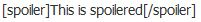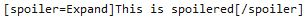Complete List of MyCodes with Examples
MyCodes are a simplified version of HTML that can be easily used to create certain effects or enhance your posts and format them properly. Here are all the MyCodes that can be currently used in this forum.

1. Bold
Code:
`[b]This text is bold[/b]`

Preview: This text is bold

2. Italic
Code:
`[i]This text is italicized[/i]`

Preview: This text is italicized

3. Underline
Code:
`[u]This text is underlined[/u]`

Preview: This text is underlined

4. Struck Out
Code:
`[s]This text is struck out[/s]`

Preview: This text is struck out

5. URL
Code:
`[url]http://www.example.com/[/url]`

Preview: http://www.example.com/

Code:
`[url=http://www.example.com/]Example[/url]`

Preview: Example

6. Email
Code:
`[email]example@example.com[/email]`

Preview: example@example.com

Code:
`[email=example@example.com]E-mail Me![/email]`

Preview: E-mail Me!

Code:
`[email=example@example.com?subject=spam]E-mail with subject[/email]`

Preview: E-mail with subject

7. "Quote"
Code:
`[quote]Quoted text will be here[/quote]`

Preview:
Quote:Quoted text will be here

8. Code
Code:
`[code]Text with preserved formatting`
[/code]

Preview:
Code:
`Text with preserved formatting`

9. Image
Code:
`[img]https://caersidi-ns.com/images/logosolas.png[/img]`

Preview:Code:
`[img=50x50]https://caersidi-ns.com/images/logosolas.png[/img]`

Preview:Code:
`[img align=center]https://caersidi-ns.com/images/logosolas.png[/img]`

Preview:Code:
`[img align=right]https://caersidi-ns.com/images/logosolas.png[/img]`

Preview:10. Color
Code:
`[color=red]This text is red[/color]`

Preview: This text is red

11. Size
Code:
`[size=3]This text is size 3[/size]`

Preview: This text is size 3

12. Font

Code:
`[font=Tahoma]This font is Tahoma[/font]`

Preview: This font is Tahoma

13. Align

Code:
`[align=center]This is centered[/align]`

Preview:
This is centered

Code:
`[align=right]This is right-aligned[/align]`

Preview:
This is right-aligned

14. List
Code:
```[list] [*]List Item #1 [*]List Item #2 [*]List Item #3 [/list]```

Preview:
• List Item #1
• List Item #2
• List Item #3
Code:
`NOTE: You can make an ordered list by using [list=1] for a numbered, and [list=a] for an alphabetical list.`

15. SpoilerPreview:
Spoiler:Preview:
Expand:

16. Box
Code:
`[box]This is a box[/box]`

Preview:
 This is a box

17. Table
Code:
```[table=Example] [th]This is my Table[/th][th]Test[/th] [tr][td]A[/td] [td]1[/td][/tr] [tr][td]B[/td] [td]2[/td][/tr] [/table]```

Preview:
[table=Example]
This is my TableTest A 1 B 2 [/table]

Code:
```Instructions: [th][/th] = Table Headers [tr][/tr] = Table Row [td][/td] = Table Data Cell```

18. Dice Roll
Code:
`[roll=low-high]`

Rolls a single dice with values between low and high, inclusive.

e.g.

Code:
`[roll=1-10]`

Potential output - Rolling 1-10: 5

Code:
`[roll=low-high+/-F]`

Rolls a single dice with values between low and high, inclusive. Adds or subtracts the given offset F.

e.g.

Code:
`[roll=1-10+5]`
Potential output  - Rolling 1-10: 2 + 5 = 7

Code:
`[roll=1-10-5]`

Potential output - Rolling 1-10: 2 - 5 = -3

Code:
`[roll=dS]`

Rolls a single dice with with values between 1 and S, inclusive.

e.g.

Code:
`[roll=d6]`

Potential output - Rolling d6: 4

Code:
`[roll=NdS]`

Rolls N dice with values between 1 and S, inclusive.

e.g.

Code:
`[roll=3d6]`

Potential output - Rolling 3d6: 4 + 2 + 6 = 12

Code:
`[roll=dS+/F]`

Rolls a single dice with values between low and high, inclusive. Adds or subtracts the given offset F.

e.g.

Code:
`[roll=d6+5]`

Potential output - Rolling d6+5: 4 + 5 = 9

Code:
`[roll=NdS+/-F]`

Rolls N dice with values between 1 and S, inclusive. Adds or subtracts the given offset F.

e.g.

Code:
`[roll=3d6+20]`

Potential output - Rolling 3d6+20: 4 + 1 + 6 + 20 = 31

Code:
`[roll=weighted list]`

Rolls a single dice with as many values as there are items in weighted list. Each item in weighted list represents how likely that index is to be selected.

e.g.
Code:
`[roll=25,25,50]`
gives 1 a 25% chance to be rolled, 2 a 25% chance, and 3 a 50% chance.

Potential output - Rolling 25,25,50: 3

19. Nation
Code:
`[nation]Lollerland[/nation]`

Preview: Lollerland

20. Region
Code:
`[region]Caer Sidi[/region]`

Preview: Caer Sidi

Forum Jump:

Users browsing this thread: 1 Guest(s)